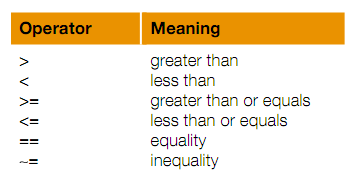## Relational expressions, MATLAB Programming

Assignment Help:

Relational Expressions:

The Conditions in if statements use expressions which are theoretically, or logically, either true or false. These expressions are termed as relational expressions, or at times Boolean or logical expressions. Such expressions can use both relational operators, that associate two expressions of compatible types, and logical operators, that operate on the logical operands.

The relational operators in MATLAB are as shown:All the concepts must be familiar, though the operators used might be different from those used in the other programming languages, or in the mathematics classes.

In particular, it is very significant to note that the operator for equality is two consecutive equal signs, not a single equal sign.

#### Ray tracing equations, i want to write a program in mat lab for optimizing ...

i want to write a program in mat lab for optimizing the design of lens help me how could i i am new user of matlab but previously i have work on C++.

#### Digging the dirt - autonomous mining robots , Digging the Dirt: Autonomous...

Digging the Dirt: Autonomous Mining Robots   Mining is a multi-billion dollar industry and a critical part of the Australian economy. Much of the day-to-day work on mining sites

30 3/4- 15 5/6

#### #title.matrix, #defining matrix using only special commands

#defining matrix using only special commands

#### Solve the system of linear equations - matlab, Problem 1.  Use Matlab to so...

Problem 1.  Use Matlab to solve the following system of linear equations: 2x + y + 3z = 1 2x + 6y + 8z = 3 6x + 8y + 18z = 5 Capture Matlab code and the result in a text fi

#### Subpixel boundary., could you help me object

could you help me object

#### Print the day of the week, how to write a program to determine the day of ...

how to write a program to determine the day of the week. using GUI.

#### Documentation - script, Documentation: It is very important that all t...

Documentation: It is very important that all the scripts be documented well, so that the people can understand what the script does and how it accomplishes that. One way of do

#### #title.algebra., #questio8a/2x=-a/8n..

#questio8a/2x=-a/8n..

#### Write a matlab program that calculates the arithmetic mean, Write a MATLAB ...

Write a MATLAB program that calculates the arithmetic mean, and the root-mean-square average for a given set of values. The output should be formatted as follows: Y